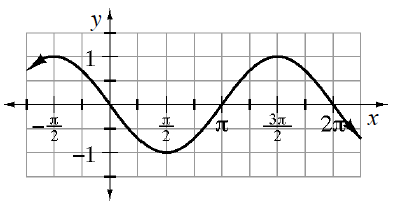### Home > INT3 > Chapter 9 > Lesson 9.2.2 > Problem9-109

9-109.Susan knows how to shift $y = \text{sin}\left(x\right)$ to get the graph at right, but she wonders if it is possible to get the same graph by shifting $y = \text{cos}\left(x\right)$.

1. Is it possible to write a cosine function for this graph?

Yes, it is.

2. If you think writing a cosine function is possible, write an equation that has cosine. If you think writing one is impossible, explain why.

The graph of $y = \text{cos} \left(x\right)$ starts at a maximum. This graph has a maximum at $x = - \frac{\pi}{2} .$

3. Adlai says, “I can get that graph without shifting to the right or left.” What equation does he write?

$y = −\text{sin}\left(x\right)$